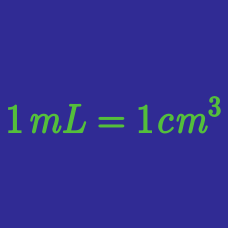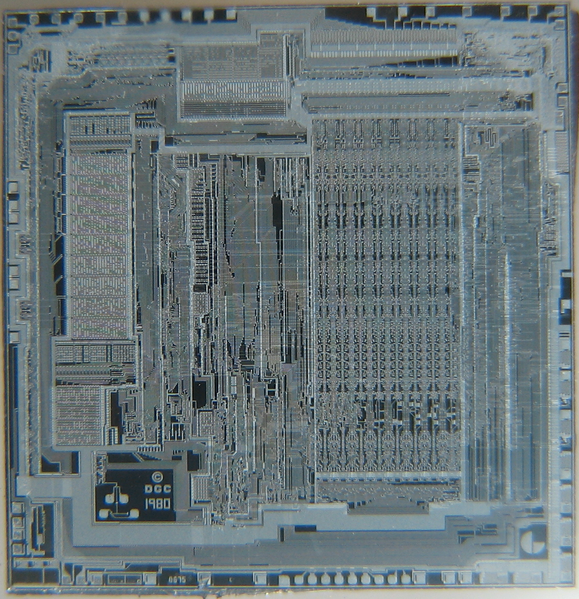Classical Mechanics

SI Multiplier PrefixesSuppose that you are told that a brand new super computer's microprocessor has a clock frequency of $3 9\text{ GHz},$ What is this frequency in hertz?

Which of the following prefixes represents $\frac{1 \text{ tera}}{1 \text{ mega}}$ of a given unit?

Which of the following prefixes represents $1 \text{ hecto } \times 1 \text{ deca }$ of a given unit?

How many gigawatts are there in $4.70 \times 10^3$ watts?

Find the expression for a particular electric power $1.27 \times 10 ^9 \text{ watts},$ using the appropriate prefix in SI unit.

×